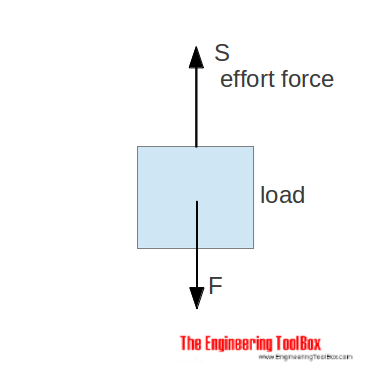Engineering ToolBox - Resources, Tools and Basic Information for Engineering and Design of Technical Applications!

# Force Ratio

## The force ratio is the load force versus the effort force.

A machine is a device that can change the magnitude and line of the action of force.Examples of machines are

A simple machine amplifies the input (effort) force to a larger output (load) force. The force ratio can be expressed as

Fr = F / S                             (1)

where

Fr = force ratio - mechanical advantage

F = load force (N, lbf)

S = effort force (N, lbf)

### Example - Force Ratio for Pulley

The effort force for a pulley with 4 ropes, no friction loss (μ = 1) and load 100 kg is calculated to 245 N.

The force ratio can be estimated to

Fr = (100 kg) (9.81 m/s2) / (245 N)

= 4

## Related Topics

• ### Mechanics

Forces, acceleration, displacement, vectors, motion, momentum, energy of objects and more.

## Related Documents

• ### Acceleration of Gravity and Newton's Second Law

Acceleration of gravity and Newton's Second Law - SI and Imperial units.
• ### Bodies Moving on Inclined Planes - Acting Forces

Required forces to move bodies up inclined planes.
• ### Centripetal and Centrifugal Acceleration Force

Forces due to circular motion and centripetal / centrifugal acceleration.
• ### Equilibrant Force

The force required to keep a system of forces in equilibrium.
• ### Force

Newton's third law - force vs. mass and acceleration.
• ### Gear Reducing Formulas

Output torque, speed and horsepower with gears.
• ### Gears

Gears effort force vs. load force.
• ### Hydraulic Force vs. Pressure and Cylinder Size

Calculate hydraulic cylinder force.
• ### Impulse and Impulse Force

Forces acting a very short time are called impulse forces.
• ### Levers

Use levers to magnify forces.
• ### Motors - Speed vs. Pulley Diameter

Pulley (Sheave) selection charts for motors ranging 850 - 3450 rpm.
• ### Movement Ratio

Machines and movement ratio (velocity ratio).
• ### Pneumatic Cylinder - Excerted Force vs. Pressure

Force excerted by pneumatic air cylinders vs. air pressure cylinder size - online calculator.
• ### Pulleys

Pulleys, blocks and tackles.
• ### Screw Jack - Effort Force vs. Load

Screw jacks and effort forces.
• ### Toggle Joint

A toggle joint mechanism can be used to multiply force.

## Engineering ToolBox - SketchUp Extension - Online 3D modeling!

Add standard and customized parametric components - like flange beams, lumbers, piping, stairs and more - to your Sketchup model with the Engineering ToolBox - SketchUp Extension - enabled for use with older versions of the amazing SketchUp Make and the newer "up to date" SketchUp Pro . Add the Engineering ToolBox extension to your SketchUp Make/Pro from the Extension Warehouse !

We don't collect information from our users. More about

## Citation

• The Engineering ToolBox (2008). Force Ratio. [online] Available at: https://www.engineeringtoolbox.com/force-ratio-d_1294.html [Accessed Day Month Year].

Modify the access date according your visit.

9.29.12